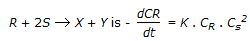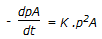# Chemical Engineering - Chemical Reaction Engineering

### Exercise :: Chemical Reaction Engineering - Section 1

16.

The rate constant of a chemical reaction decreases by decreasing the

 A. pressure B. concentration of reactants C. temperature D. duration of reaction

Explanation:

No answer description available for this question. Let us discuss.

17.

Reaction rate equation for the reaction,. If S at is present in large excess, what is the order of this reaction?

 A. zero B. one C. two D. three

Explanation:

No answer description available for this question. Let us discuss.

18.

Rate of a gaseous phase reaction is given by,. The unit of rate constant is

 A. (atm)-1 B. (hr)-1 C. (atm)-1.(hr)-1 D. atm.(hr)-1

Explanation:

No answer description available for this question. Let us discuss.

19.

__________ is the response curve for a step input signal from a reactor.

 A. S-curve B. C-curve C. I-curve D. none of these

Explanation:

No answer description available for this question. Let us discuss.

20.

'If the catalyst pore size is small in comparison with the mean free path, collisions with the pore wall controls the process'. The diffusivity under this condition is called 'Knudsen diffusivity', which is affected by the

 A. pressure B. temperature C. both (a) & (b) D. neither (a) nor (b)